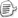概述:对于所有直接操作内存的程序员来说,数据对齐都是很重要的问题.数据对齐对你的程序的表现甚至能否正常运行都会产生影响.就像本文章阐述的一样,理解了对齐的本质还能够解释一些处理器的"奇怪的"行为.Figure 1. 程序员是如何看内存的Figure 2. 处理器是如何看内存的Figure 3. 单字节存取Figure 4. 双字节存取Figure 5. 四字节存取Figure 6. 处理器如何处理非对齐内存地址

PowerPC各取所长.目前所有的PowPC都硬件支持非对齐的32位整型的存取.虽然牺牲掉了一部分性能,但这些损失在逐渐减少.

Listing 1. 每次处理一个字节

void Munge8( void *data, uint32_t size ){
uint8_t *data8 = (uint8_t*)data;
uint8_t *data8End = data8 +size;
while( data8 != data8End ){
*data8++ = -*data8;
}
}

Listing 2.每次处理2个字节

void Munge16( void *data, uint32_t size ){
uint16_t *data16 = (uint16_t*)data;
uint16_t *data16End = data16 + (size>> 1); /* Divide size by 2. */
uint8_t *data8 = (uint8_t*)data16End;
uint8_t *data8End = data8 + (size& 0x00000001); /* Strip upper 31 bits. */
while( data16 != data16End ){
*data16++ = -*data16;
}
while( data8 != data8End ){
*data8++ = -*data8;
}
}Figure7. 单字节存取 vs.双字节存取

Listing 3. 每次处理4个字节

void Munge32( void *data, uint32_t size ){
uint32_t *data32 = (uint32_t*)data;
uint32_t *data32End = data32 + (size>> 2); /* Divide size by 4. */
uint8_t *data8 = (uint8_t*)data32End;
uint8_t *data8End = data8 + (size& 0x00000003); /* Strip upper 30 bits. */
while( data32 != data32End ){
*data32++ = -*data32;
}
while( data8 != data8End ){
*data8++ = -*data8;
}
}Figure8. 单字节vs.双字节vs.四字节存取

Listing 4.每次处理8个字节

void Munge64( void *data, uint32_t size ){
double *data64 = (double*)data;
double *data64End = data64 + (size>> 3); /* Divide size by 8. */
uint8_t *data8 = (uint8_t*)data64End;
uint8_t *data8End = data8 + (size& 0x00000007); /* Strip upper 29 bits. */
while( data64 != data64End ){
*data64++ = -*data64;
}
while( data8 != data8End ){
*data8++ = -*data8;
}
}

Munge64处理对齐的缓冲区需要39085微秒--大约比对齐的Munge32快10%.但是,在非对齐缓冲区上的处理时间是让人惊讶的1841155微秒--比对齐的慢了两个数量级,慢了足足4610%.Figure 9. 多字节存取对比Figure 10. 多字节存取对比 #2Figure10. 多字节存取对比 #3

The End.posted on 2014-11-20 14:31  MerlinJ  阅读(718)  评论(0编辑  收藏